# Robotics and Automation Proof Reading Services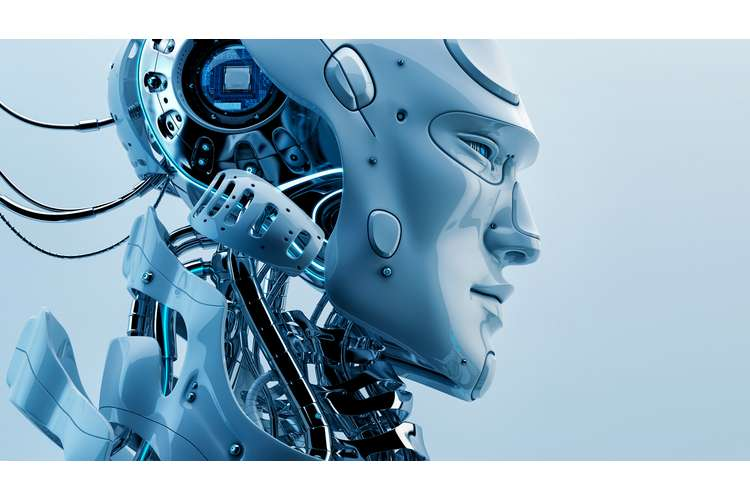## Abstract

The aim of this report is to establish understanding about the feedback control system of the robotic arm and its function within the system. PID controller is used for the feedback system n this report and further explain its transfer function for the clarification. Feedback system is lifeline for the control system of any machinery and in robots it is essential because it alters the power of actuators and help in moving towards.

### Introduction

Research suggested that a rapid progress is observed in robotics and it leads to the huge expectations for the robots which is applied in various field such as industrial robots, home robots, and guard robots. in This report an unique technique is used to acute the verities of joints and basic problem in robotic arm is to recognize the various forces and torques. So a closed-loop PID controlled is used to resolve this problem. This paper concerned about the implementation of feedback control system in Robots for the better movement of sensor against the test object. It will increase the accuracy of robot in terms of their interaction to the external environment. PID controller generally consists of proportional, integral, and derivative circuitry but in this paper the whole PID controller is instrumented and used for further simplification. The transfer function is the basic mathematical expression that can be related to the input and output variables by implementing Laplace transforms.

### Challenges

Problems that may arise during control system of robot are outlined below (Åkerberg, Gidlund, and Björkman, 2011):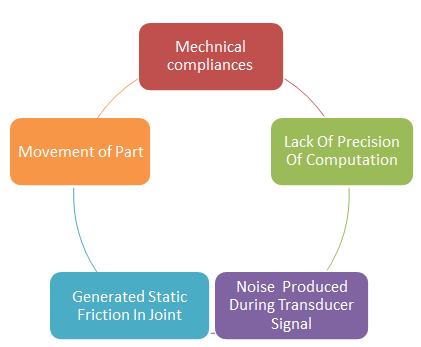Figure 1 – Challenges That May Arise

### Feedback Control System In Robot

Feedback control system is very useful while designing control system i.e. control the output of the system. It is the matter of concern when the output deviates from standard value so to steer this output feedback control system is used. Variable is required to be controlled in feedback control system and the measure at feedback system is done by comparing it with the set point i.e. predetermined. (Lunze and Lehmann, 2010) The feedback controller takes the error and further decides what should be done with those errors by compensating variable and further removes the generated error. A schematic diagram of feedback control system is given below: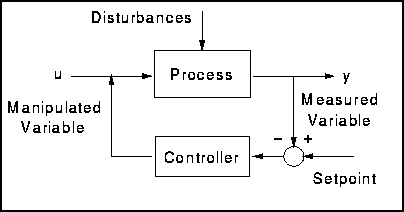Figure 2 – feedback control system

(Islamand Liu, 2011)In Robots for moving and controlling robots actuators is used. Actuator is a mechanical device which is responsible for the moving of parts within the body of machines and it generally requires a control signal and source of energy. So the movement of actuator is decided by some factors i.e. control system sends signal to actuator and those factors decide what will happen with those given control system.( Friedland, B., 2012) The main function of feedback control system is to detect the output of actuator so overall control system can correct for the external environment. Some sensors are used for the feedback control system like Encoders, Tachometers, and Accelerometers etc. some the function of the feedback control system in robotics outline below:

1.It determines the position of rotor

2.It determines the speed of rotor from one source to another

3.Position sensor is used to monitor the position of arm of robots

4.It alters the power or signal given to the actuator so movement will get desired path

5.Errors are corrected by system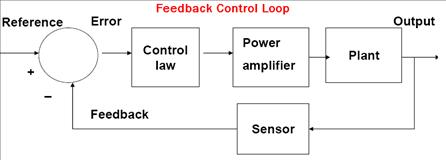Figure 3 – Feedback control loop in robots

It is the basic figure of control system in robots which contains input, output, and feedback. Feedback is recorded by some sensors shown in figure 3 i.e. directly connected to link before output. Before processing to the output the action is verified and signal reaches to the feedback sensors which further alerted the power and correction of error is done. Feedback control system has two basic objectives which are outlined below (Arrieta, Visioli, and Vilanova, 2010):

a.Servo control loopresponds to the deviation in set point i.e. there is any change in set point so this loop is responsible to respond it. Servo control is shown in figure 4.

b.A regulatory control loopresponds to the deviated input values i.e. respond to the change in input value. Schematic diagram for regulatory control loop is shown in figure 5.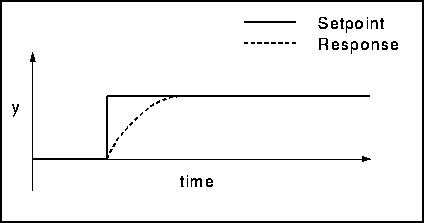Figure 4 – servo control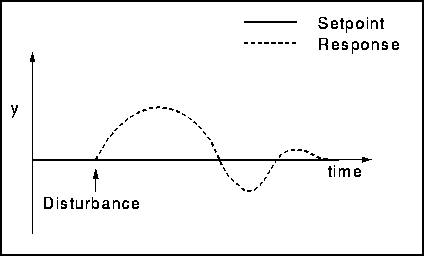Figure 5 – Regulatory control

### Transfer Function

The transfer function of the robotic arm can be approximated by establishing equation using following variables given below: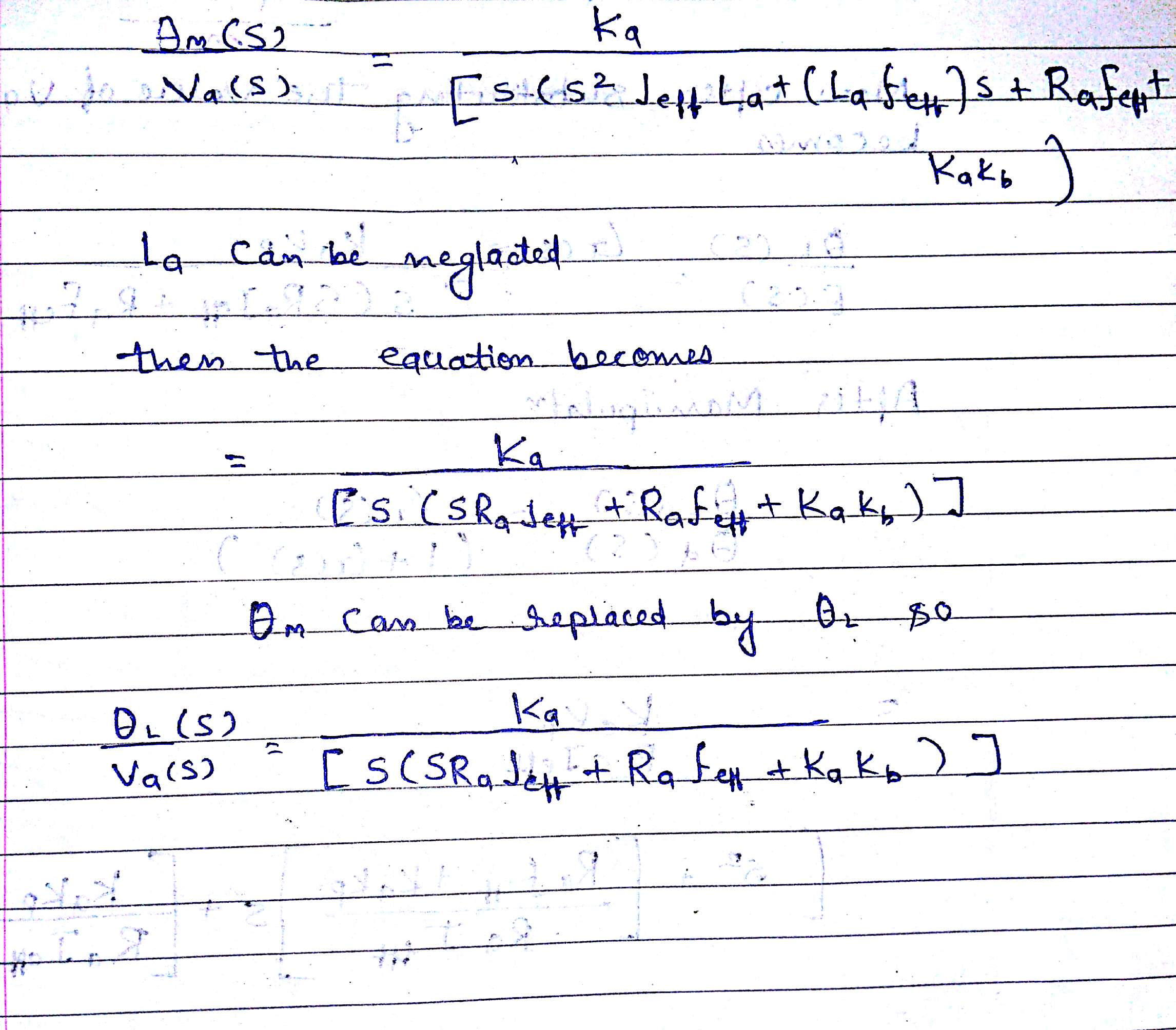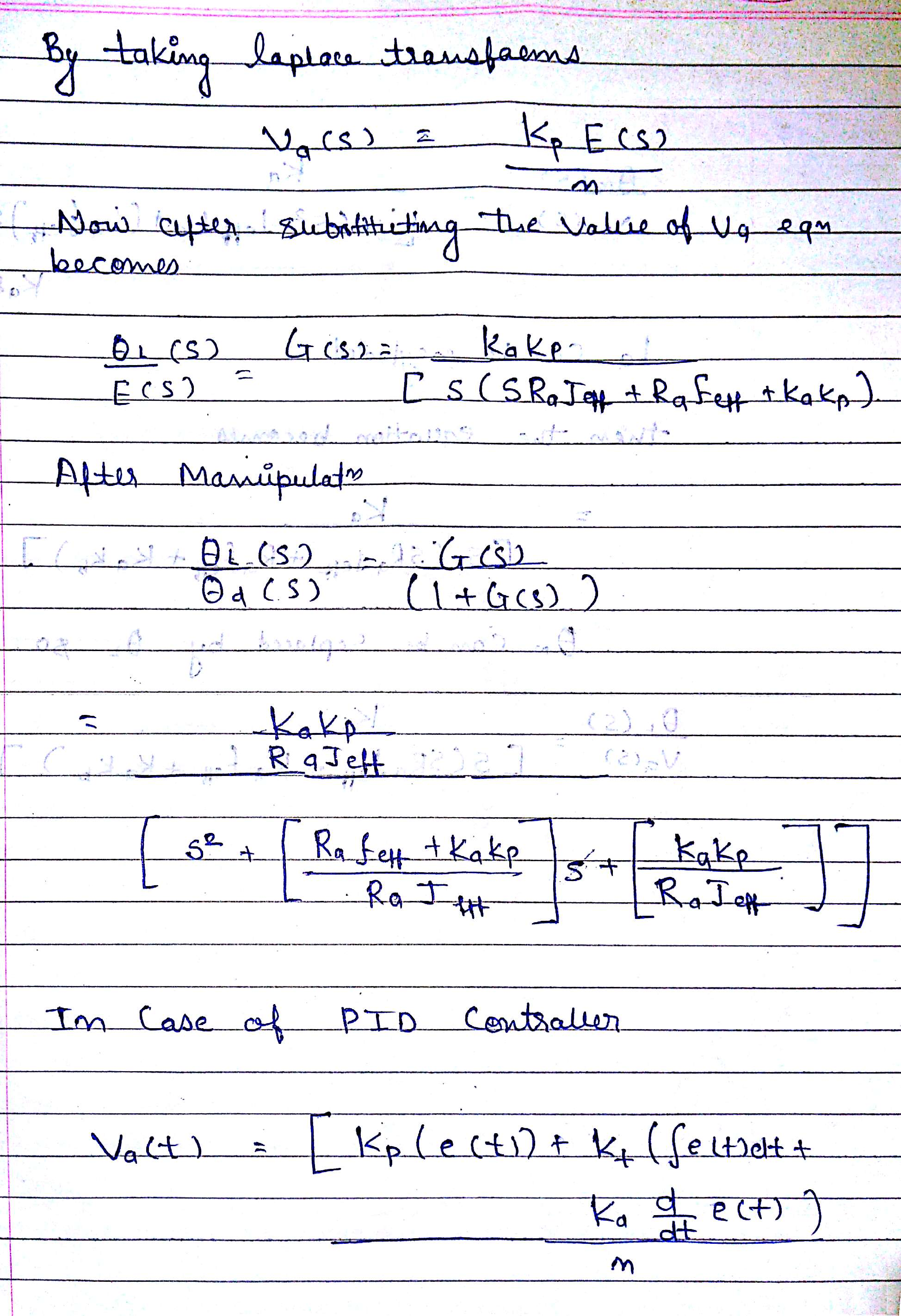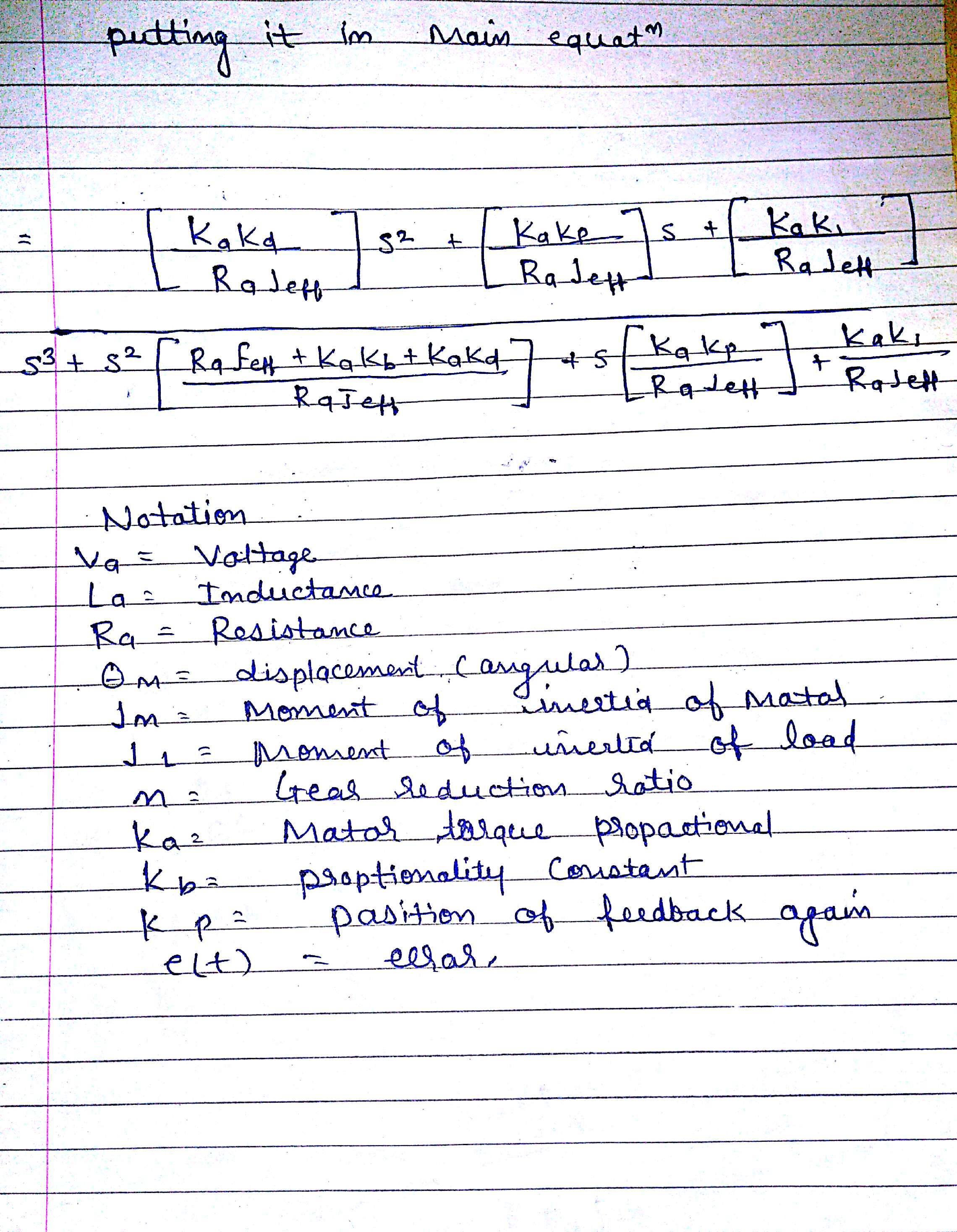### Design Of Controller

A controller needs to calculate required path correction to react the measured forces by sensor and for this offset of the path is required. In figure 8 below a close loop system is shown where “r” Is the set point value, “y” is the variable i.e. process variable, “C” is the controller, and “P” is the robot system. As to make it effective and useful PID controlled is choose because it will provide simplicity and robustness to the system.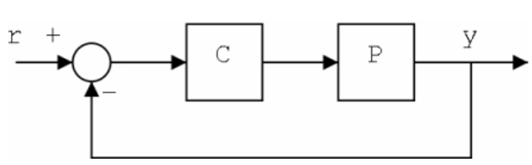Figure 8 – Closed loop system

PID controllers has a set point “r”, “u” as a output, offset “y”, and Gains i.e. PID gains “p”, “I”, and “D” as shown in figure 9 . But mostly in case of robotics it contains manual control and output limiter as well i.e. the output limiter alters the value of output signals that may be achieved. Further a Gain scheduler is implemented which compares the input value to the threshold values i.e. it consists of the setting of threshold as well as P, I, D setting. Schematic diagram of gain schedule is shown in figure 10(Ayala and dos Santos Coelho, 2012).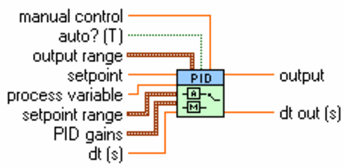Figure 9 – PID controller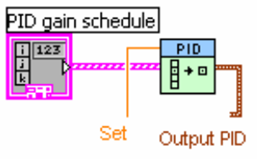Figure 10 – Gain scheduler

A combination of proportional, integral, and derivative action is known as PID Controller. PID controller receives input from the sensor and also accepts the output actuator i.e. set variable. Further computation process takes place by the combination of integral, proportional, and derivative responses to generate the response output for actuators. A typical process of PID controller is shown in figure 1 where process variable is maintained at an appropriate level.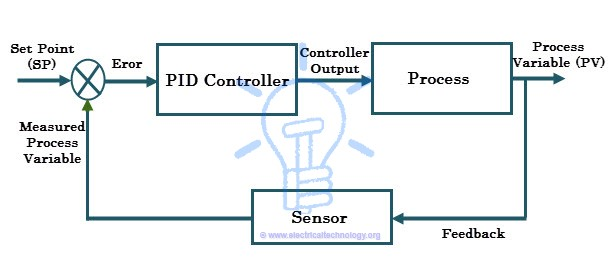Figure 11– Process of PID controller

### References

1.Lunze, J. and Lehmann, D., 2010. A state-feedback approach to event-based control. Automatica, 46(1), pp.211-215.

2.Islam, S. and Liu, P.X., 2011. Robust adaptive fuzzy output feedback control system for robot manipulators. IEEE/ASME Transactions onmechatronics, 16(2), pp.288-296.

3.Friedland, B., 2012. Control system design: an introduction to state-space methods. Courier Corporation (online) available at http://s3.amazonaws.com/academia.edu.documents/39219970/Control_System_Design_-_An_Introduction_to_State-Space_Methods_-_Bernard_Friedland_Dover_Publications.pdf?AWSAccessKeyId=AKIAIWOWYYGZ2Y53UL3A&Expires=1490180146&Signature=H0aEmGWo%2FNM8rFfxAY5PcU3lw2E%3D&response-content-disposition=inline%3B%20filename%3DControl_System_Design_-_An_Introduction.pdf last accessed on 22/03/2017.

4.Åkerberg, J., Gidlund, M. and Björkman, M., 2011, July. Future research challenges in wireless sensor and actuator networks targeting industrial automation. In Industrial Informatics (INDIN), 2011 9th IEEE International Conference on (pp. 410-415). IEEE.

5.Arrieta, O., Visioli, A. and Vilanova, R., 2010. PID autotuning for weighted servo/regulation control operation. Journal of Process Control, 20(4), pp.472-480.

6.Salih, A.L., Moghavvemi, M., Mohamed, H.A. and Gaeid, K.S., 2010, May. Modelling and PID controller design for a quadrotor unmanned air vehicle. In Automation Quality and Testing Robotics (AQTR), 2010 IEEE International Conference on (Vol. 1, pp. 1-5). IEEE.

7.Chang, W.D. and Shih, S.P., 2010. PID controller design of nonlinear systems using an improved particle swarm optimization approach. Communications in Nonlinear Science and Numerical Simulation, 15(11), pp.3632-3639.

8.Ayala, H.V.H. and dos Santos Coelho, L., 2012. Tuning of PID controller based on a multiobjective genetic algorithm applied to a robotic manipulator. Expert Systems with Applications, 39(10), pp.8968-8974.